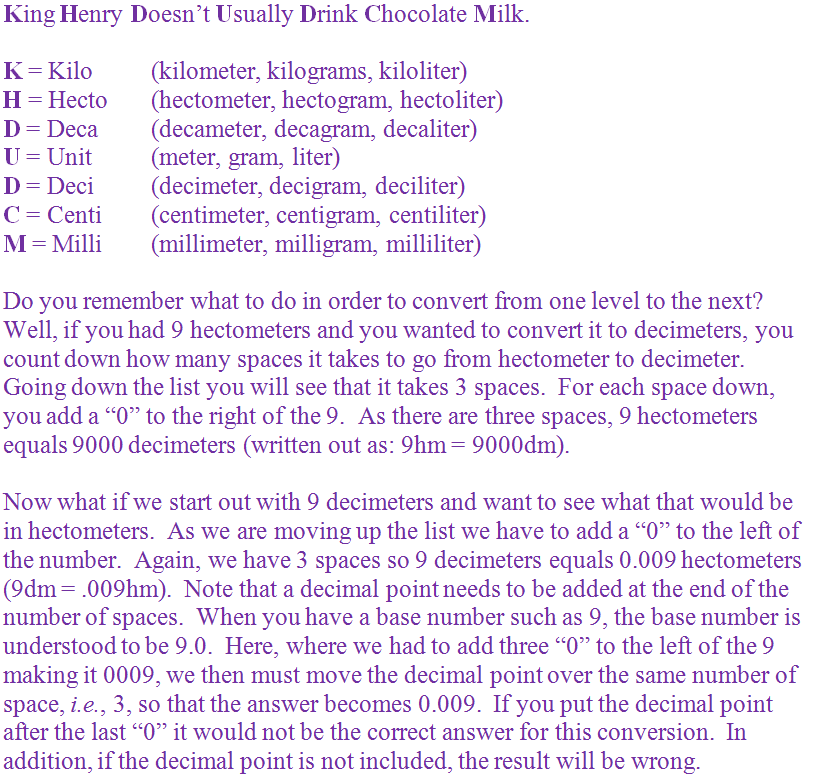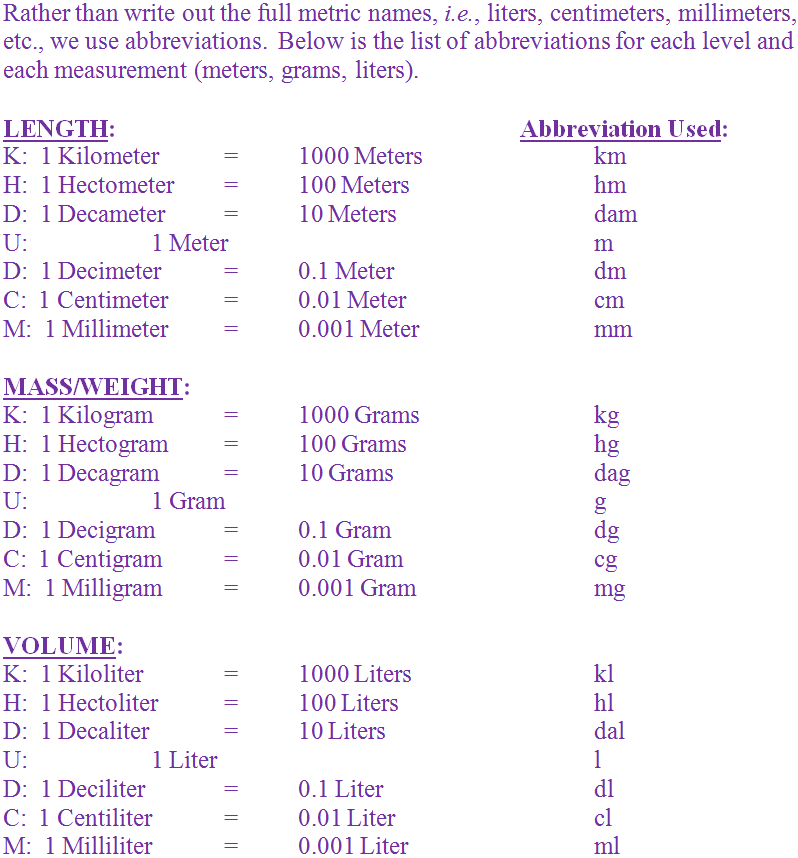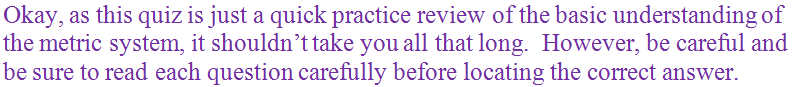The metric system is a set of standardized units of measurement based on the number 10.

# Measurements - Metric System - Review of the Basics

This Math quiz is called 'Measurements - Metric System - Review of the Basics' and it has been written by teachers to help you if you are studying the subject at middle school. Playing educational quizzes is a fabulous way to learn if you are in the 6th, 7th or 8th grade - aged 11 to 14.

It costs only \$12.50 per month to play this quiz and over 3,500 others that help you with your school work. You can subscribe on the page at Join Us

Does the “metric system” sound familiar to you? You might remember it from your math classes. The metric system is a set of standardized units of measurement based on the number 10. It is the system that most of the world works off of. Here in the United States, and only two other countries, i.e., Liberia and Burma, we use what is called either the American or the English system.

As the English system is far more complicated, learning to use the metric system is rather an easy challenge. All the same, it does take some retraining of the mind to become comfortable using this new system. However, learning it is crucial and essential as you will encounter it in many areas of life. In fact, you already encounter it.

For example, in measurement of volume, you buy a 2 liter bottle of soda. You don’t buy a quart of soda or a pint of soda or even a gallon of soda. Nope – you buy “liters” of soda. A liter is the measurement of volume in the metric system. In addition to liters, the metric system includes meters to measure length and distance and, finally, grams to measure mass or weight.

As you may also recall, there is a little catchy phrase to use that will help you to remember the order of the metric system. If you have forgotten it, you can see it again below.

Go straight to Quiz1.
This is the metric measurement of mass and/or weight.
Pounds
Grams
Meters
Liters
In the metric system, grams refer to the measurement of mass and/or weight. Answer (b) is the correct answer
2.
The standardized method for math in the United States is the ________.
Burma system
Universal system
English system
Liberia system
The standardized method of math in the United States is the English system (also referred to as the American system). Answer (c) is the correct answer
3.
In the United States, milk is purchased in _______.
liters
grams
meters
gallons
Here in the United States, milk is purchased in gallons. However, soda is purchased in liters. Answer (d) is the correct answer
4.
If you were asked to convert milligrams to decagrams, how many spaces apart would that be?
4
3
6
5
To convert milligrams to decagrams you would have to count up 4 spaces. Answer (a) is the correct answer
5.
If you were asked to convert kiloliters to centiliters, how many spaces apart would that be?
3
5
7
4
To convert kiloliters to centiliters you would have to count down 5 spaces. Answer (b) is the correct answer
6.
When counting up the metric list, you must do what to your base number?
Multiply the base number by the number of spaces
Divide the base number by the number of spaces
Put the required number of zeros to the right of the base number
Put the required number of zeros to the left of the base number and add a decimal point after the same number of spaces
When counting up the metric list, you must add the required number of zeros to the left of the base number. After the required number of zeros, you must then add in a decimal point the same number of required spaces to the left of the base number. Answer (d) is the correct answer
7.
This is the metric measurement of length and distance.
Feet
Liters
Meters
Grams
In the metric system, length and distances are measured in meters. Answer (c) is the correct answer
8.
To measure the volume of an area you must have 3 reference points. In the metric system, the volume is measured in _____.
liters
weight
mass
meters
To measure the volume of an area you must have 3 reference points. In the metric system, the volume is measured in liters. Answer (a) is the correct answer
9.
Which of the following is NOT part of the metric system?
Decagram
Diameter
Centiliter
Kilometer
A diameter is not part of the metric system. It is a measurement of a circle. Answer (b) is the correct answer
10.
When counting down the metric list, you must do what to your base number?
Add the same number of zeros to the left of the number as the number of spaces
Divide the base number by the number of spaces
Multiply the base number by the number of spaces
Add the required number of zeros to the right of the base number
When counting down the metric list, you must add the required number of zeros to the right of the base number. Answer (d) is the correct answer
Author:  Christine G. Broome